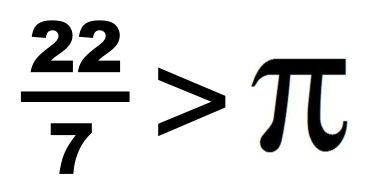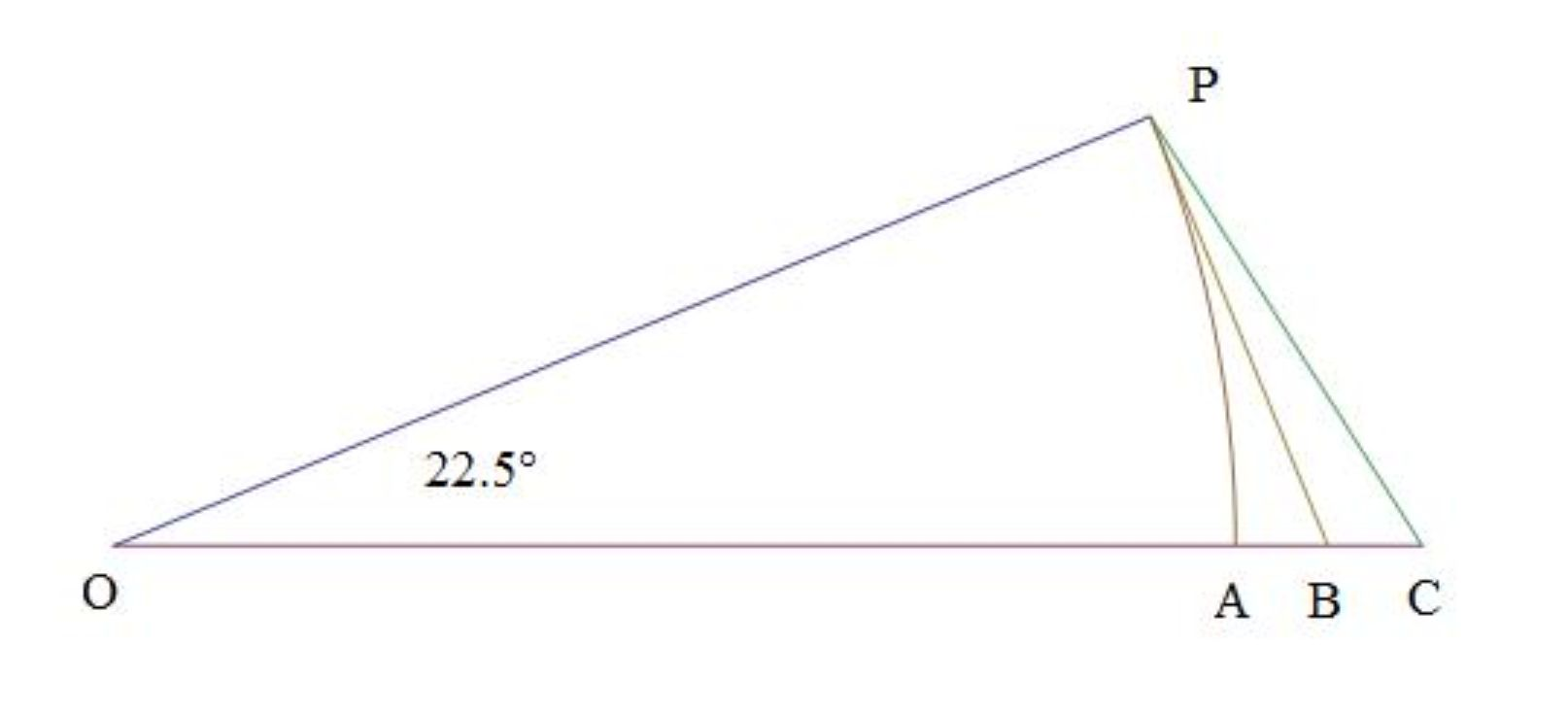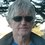# Updating Archimedes' Method of Approximating πA popular math problem is proving that $22/7$ exceeds $\pi$, without resorting to known values of $\pi$ or approximations by calculator. One method uses certain definite integrals which work out to $22/7$ $-\pi$ which is known to be greater than $0$. But back around 250 BC, Archimedes was the first to prove that $22/7$ exceeds $\pi$, by successive use of the trigonometric identity

$Tan(2x)=\dfrac { 2Tan(x) }{ 1-{ (Tan(x)) }^{ 2 } }$

Starting with the regular hexagon, and doubling the number of sides until he reached a regular polygon of $96$ sides, he was able to show that $22/7$ does indeed exceed $\pi$. Unfortunately, he relied on a series of inequalities in his computations, the notes of which are lost, so we have no complete proof left to us from his works. So, here’s an updated version of his approach that will be an exact proof, beginning with the following diagramwhere

$\angle OPB=90°$
$OP=OA=1$
$OB=Sec(\dfrac { 45° }{ n } )$
$OC=(\dfrac { a }{ b } )(\dfrac { 1 }{ 4n } )Csc(\dfrac{45°}{n})$

In this diagram shown, $n=2$ and $\dfrac { a }{ b } =\dfrac { 25 }{ 7 }$, a very crude apprximation of $\pi$. $\dfrac { a }{ b }$ is selected so that $OC>OB$, so that it is clear that the areas are

$\left[ OPC \right] >\left[ OPB \right] >\left[ OPC \right]$

That is

$(\dfrac { 1 }{ 8n } )(\dfrac { a }{ b } )>(\dfrac { 1 }{ 2 } )Tan(\dfrac { 45° }{ n } )>(\dfrac { 1 }{ 8n } )\pi$

so that if we can prove that for some sufficiently large $n$

$(\dfrac { 1 }{ 4n } )(\dfrac { a }{ b } )>Tan(\dfrac { 45° }{ n } )$

then we've proven that

$\dfrac { a }{ b } >\pi$

Now but there is a delightfully useful trigonometric identity to use for this purpose, which Archimedes didn't have

$Tan(nx)=F(n,Tan(x))=i\dfrac { { (1-iTan(x)) }^{ n }-{ (1+iTan(x)) }^{ n } }{ { (1-iTan(x)) }^{ n }+{ (1+iTan(x)) }^{ n } }$

If $(\dfrac { 1 }{ 4n } )(\dfrac { a }{ b } )>Tan(\dfrac { 45° }{ n } )$, then

$F(n,(\dfrac { 1 }{ 4n } )(\dfrac { a }{ b } ))>F(n,Tan(\dfrac { 45° }{ n } ))$, (a little handwaving here), but since

$F(n,Tan(\dfrac { 45° }{ n } ))=1$, we have

$F(n,(\dfrac { 1 }{ 4n } )(\dfrac { a }{ b } ))>1$

So, for $\dfrac { a }{ b } =\dfrac { 22 }{ 7 }$, all we have to do is to show that for some sufficiently large $n$, let's say $n=24$, which corresponds to the regular $96$ sided polygon Archimedes used

$F(n,(\dfrac { 1 }{ 4n } )(\dfrac { a }{ b } ))=F(24,(\dfrac { 1 }{ 96 } )(\dfrac { 22 }{ 7 } ))>1$

Using that trigonometric identity above, the exact final rational fraction is

$\dfrac { 3070617780371250623172531488874792422422762969087263790473600 }{3070399174588386853835740065608415583799863505007168821897761 }$

Note that the first $6$ digits of the numerator and denominator are

$307061>307039$

which conclusively and exactly proves that $22/7$$>\pi$, without resorting to approximations, without using known computed values of $\pi$, nor infinite series, nor infinite products, nor infinite continued fractions.

Going further, we can let $n=1557$, corresponding to a regular polygon of $6228$ sides, and let $a/b=355/113$, another famous and very accurate approximation. We end up with a rational fraction with a numerator and a denominator both $9105$ digits long, the first $12$ digits of each being

$196554866601 > 196554866571$

thus proving that $355/113>\pi$ as well.

Addendum: Note that as $n\rightarrow \infty$

$Tan(x)=Tan(n(\dfrac { x }{ n } ))=F(n,Tan(\dfrac { x }{ n } ))\approx F(n,(\dfrac { x }{ n } ))$

$F(n,(\dfrac { x }{ n } ))=i\dfrac { { (1-\dfrac { ix }{ n } ) }^{ n }-{ (1+\dfrac { ix }{ n } ) }^{ n } }{ { (1-\dfrac { ix }{ n } ) }^{ n }+{ (1+\dfrac { ix }{ n } ) }^{ n } } =i\dfrac { { e }^{ -ix }-{ e }^{ ix } }{ { e }^{ -ix }+{ e }^{ ix } } =Tan(x)$

which shows the relationship between the exponential form of the $Tan(x)$ function and the trigonometric identity given above for $Tan(nx)$Note by Michael Mendrin
6 years, 11 months ago

This discussion board is a place to discuss our Daily Challenges and the math and science related to those challenges. Explanations are more than just a solution — they should explain the steps and thinking strategies that you used to obtain the solution. Comments should further the discussion of math and science.

When posting on Brilliant:

• Use the emojis to react to an explanation, whether you're congratulating a job well done , or just really confused .
• Ask specific questions about the challenge or the steps in somebody's explanation. Well-posed questions can add a lot to the discussion, but posting "I don't understand!" doesn't help anyone.
• Try to contribute something new to the discussion, whether it is an extension, generalization or other idea related to the challenge.

MarkdownAppears as
*italics* or _italics_ italics
**bold** or __bold__ bold
- bulleted- list
• bulleted
• list
1. numbered2. list
1. numbered
2. list
Note: you must add a full line of space before and after lists for them to show up correctly
paragraph 1paragraph 2

paragraph 1

paragraph 2

[example link](https://brilliant.org)example link
> This is a quote
This is a quote
    # I indented these lines
# 4 spaces, and now they show
# up as a code block.

print "hello world"
# I indented these lines
# 4 spaces, and now they show
# up as a code block.

print "hello world"
MathAppears as
Remember to wrap math in $$ ... $$ or $ ... $ to ensure proper formatting.
2 \times 3 $2 \times 3$
2^{34} $2^{34}$
a_{i-1} $a_{i-1}$
\frac{2}{3} $\frac{2}{3}$
\sqrt{2} $\sqrt{2}$
\sum_{i=1}^3 $\sum_{i=1}^3$
\sin \theta $\sin \theta$
\boxed{123} $\boxed{123}$

Sort by:

My favorite proof is this funny integral. $0 < \int_0^1 \frac{x^4(1-x)^4}{1+x^2} \,\mathrm dx = \frac{22}7 - \pi$

- 6 years, 10 months ago

Well, it's true that it's hard to beat that one as "something that can be done on the back of an envelope". A large envelope anyway.

- 6 years, 10 months ago

Dude, you just published the solution of my problem! Why?!

- 6 years, 10 months ago

Probably because she didn't know you posted this as a problem?

- 6 years, 10 months ago

Like you said Sir. I did not know that. Besides, I already knew this problem long time ago. This problem is a problem in Putnam competition.

- 6 years, 10 months ago

All right, but now she knows.

- 6 years, 10 months ago

Gimme a break!? How do I know that?? As Mr. Mendrin said, I did NOT know that. Why didn't you comment by inserting your problem + link direction instead of link only?? (¬‿¬)

- 6 years, 10 months ago

Also, can you tell me how to use + link that you are telling?

- 6 years, 10 months ago

Use [your problem](link direction)

- 6 years, 10 months ago

All right, since you now know, can you please delete your comment?

- 6 years, 10 months ago

Valentina, actually, I didn't mind you posting the solution to that classic definite integral. That was the one I was thinking about when I referred to them at the beginning of this short paper. But it's not the only one possible. Maybe you can post another that isn't the same as Avineil's posted problem?

- 6 years, 10 months ago

Right now, I have no idea. If something crosses to mind, I will post it here.

- 6 years, 10 months ago

Eh, but you know, at the point where I said, "a little handwaving here", there's actually more that needs to be said before this really becomes a complete proof. But this tiny detail will take up too much space to resolve.

- 6 years, 11 months ago

That's really something, u know!! Thankx for posting it.. Quite enlightening. :-)

- 6 years, 11 months ago

@Michael Mendrin , Your note inspired my next sum!

Integrate It! Part-V

https://brilliant.org/community-problem/integrate-it-part-v/?group=DvYD57CYrdYl&just_created=true

- 6 years, 11 months ago

Wow.

- 6 years, 11 months ago

I know right!? :D

- 6 years, 11 months ago